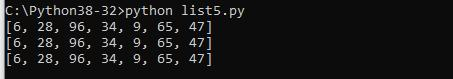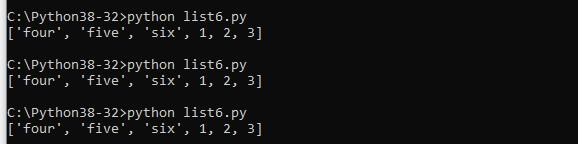Home » Python lists : more examples

# Python lists : more examples

In this article we look at more functions and methods associated with lists, this is a follow on from our first part – Python Lists : the basics

### Length, maximum and minimum values of a list

First lets cover the following built in functions

len(list) the total length of the list.
max(list) Returns item from the list with maximum value
min(list) Returns item from the list with minimum value

Lets look at an example

```#!/usr/bin/python
​
numbers = [6, 28, 96, 34, 9, 65, 47];
print (numbers)
#get the length
print ("list length : ", len(numbers))
#print the minimum value
print ("min value : ", min(numbers))
#print the maximum value
print ("max value : ", max(numbers))```

Save this as list4.py and run this example from the command line with python list4.py and you should see something like this

### Copy a list

There are a couple of ways of doing this you can use the copy() method and the built-in method list(). Lets look at an example

```#!/usr/bin/python

numbers1 = [6, 28, 96, 34, 9, 65, 47];
print(numbers1)
numbers2 = numbers1.copy()
print(numbers2)
numbers3 = list(numbers2)
print(numbers3)```

The output when this is run will be### Join two Lists

Again there are a couple of ways to copy two lists

You can use the + operator, you can append all the items from list2 into list1, one by one and you can use the extend() method to add elements from one list to another list.

Lets look at some examples

#### using the + operator

```list1 = ["four", "five" , "six"]
list2 = [1, 2, 3]

list3 = list1 + list2
print(list3)```

append all the items example

```#!/usr/bin/python

list1 = ["four", "five" , "six"]
list2 = [1, 2, 3]

for x in list2:
list1.append(x)

print(list1)
use the extend() method

#!/usr/bin/python

list1 = ["four", "five" , "six"]
list2 = [1, 2, 3]

list1.extend(list2)
print(list1)```

All of these examples when run will output the same result – something like this### Sort a list

You can use the sort() method which will sort the list ascending by default.

list.sort(reverse=True|False, key=optionalFunction)

reverse – Optional. reverse=True will sort the list descending. Default is reverse=False
key – Optional. A function to specify the sorting criteria(s)

Lets look at an example

```#!/usr/bin/python

numbers = [6, 28, 96, 34, 9, 65, 47];
numbers.sort(reverse=True)
print(numbers)
numbers.sort()
print(numbers)```

This is what you should see### Count list items and reverse a list

The count() method returns the number of elements with a specific value.
The reverse() method reverses the sorting order of the elements.

Lets look at an example

```#!/usr/bin/python

numbers = [6, 5, 4, 5, 6, 3, 2, 1, 3, 2, 6];
print(numbers)
x = numbers.count(6)
print(x)
numbers.reverse()
print(numbers)```

This is what you should see### Check if a number is in the list

You can use the in keyword to check if an item is in the list. Here is an example

```#!/usr/bin/python

numbers = [6, 28, 96, 34, 9, 65, 47];
if 6 in numbers:
print("the number 6 is in the list")

if 12 in numbers:
print("the number 12 is in the list")
else:
print("the number 12 is missing")```

### Looping through a list

You can easily loop through all the items in a list using a for loop

```#!/usr/bin/python

numbers1 = [6, 28, 96, 34, 9, 65, 47];
for x in numbers1:
print(x)```

Save this as list6.py, run it from the command line using python list6.py and you should see something like this0 comment

#### You may also like

This website uses cookies to improve your experience. We'll assume you're ok with this, but you can opt-out if you wish. Accept Read More